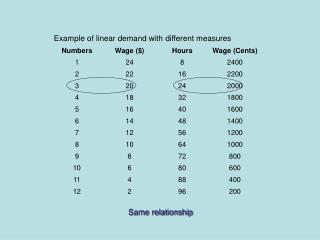DownloadDownload PresentationSame relationship

# Same relationship

Download Presentation## Same relationship

- - - - - - - - - - - - - - - - - - - - - - - - - - - E N D - - - - - - - - - - - - - - - - - - - - - - - - - - -
##### Presentation Transcript

1. Same relationship

2. =(ΔW)/(ΔN)

3. =(ΔW)/(ΔN) Slopes are sensitive to the units Need a unit free measure of labor demand sensitivity

4. Computing the elasticity Own wage elasticity of demand for labor: Percentage change in labor demand caused by a 1% change in the wage • N: labor • W: wage

5. Computing the elasticity Own wage elasticity of demand for labor: Percentage change in labor demand caused by a 1% change in the wage Units cancel

6. >9.5 7<

7. ΔW=2 ΔN=1 =(1/9.5) / (2/7) = |-.368|

8. >76 700<

9. ΔW=200 ΔN=8 =(8/76) / (200/700) = |-.368|

10. Relationship between demand slope and elasticity Slope of demand curve is (ΔW)/(ΔN)

11. Relationship between demand slope and elasticity Elasticity = |(1/slope)*(W/N)|

12. Relationship between demand slope and elasticity As the demand slope gets bigger , the demand elasticity gets smaller Elasticity = |(1/slope)*(W/N)| => W 4 3 2 1 N

13. Relationship between demand slope and elasticity Extremes: 3: slope = 0 ηNN Elasticity = |(1/slope)*(W/N)| W 4 3 2 1 N

14. Relationship between demand slope and elasticity Extremes: 3: slope = 0 ηNN Elasticity = |(1/slope)*(W/N)| W 4 Perfectly E lastic 3 2 1 N

15. Relationship between demand slope and elasticity Elasticity = |(1/slope)*(W/N)| W 4 Extremes: 4: slope = - ηNN = 0 3 2 1 N

16. Relationship between demand slope and elasticity Elasticity = |(1/slope)*(W/N)| Extremes: 4: slope = - ηNN = 0 W 4 Perfectly nelastic 3 2 1 N

17. Relationship between demand slope and elasticity Elasticity = |(1/slope)*(W/N)| W 4 Relatively Inelastic Demand 3 Relatively Elastic Demand 2 1 N

18. If you are a union representative, which demand curve would you want? W 4 Aim: Maximize the wage bill = W*N 3 2 1 N

19. Labor demand elasticity and the wage bill W W1 Labor demand: N: number of workers; W: Wage W0 Demand N N1 N0 Wage Bill = W*N; Change in wage bill = W1N1 – W0N0

20. Labor demand elasticity and the wage bill W Relatively Inelastic Demand W1 W0 Relatively Elastic Demand N N1 N2 N0 Change in wage bill Relatively Inelastic demand, Δ(W*N) = W1N2 – W0N0 Relatively Elastic demand, Δ(W*N) = W1N1 – W0N0

21. Labor demand elasticity and the wage bill W Relatively Inelastic Demand W1 W0 Relatively Elastic Demand N N1 N2 N0 Change in wage bill Relatively Inelastic demand, Δ(W*N) = W1N2 – W0N0 Relatively Elastic demand, Δ(W*N) = W1N1 – W0N0 Bigger

22. Precise relationship between demand elasticity and the wage bill ED = Elasticity of demand = % change in employment % change in wage 0 < ED < 1: inelastic demand ED = 1: unitary elastic demand ED > 1: elastic demand Wage increase with inelastic demand will raise the wage bill Wage increase with elastic demand will lower the wage bill

23. EXAMPLE ED = Elasticity of demand = 0.3 < 1, inelastic % change in employment = 3% % change in wage = 10% W1 = W0 (1.10) N1 = N0 (0.97) Change in wage bill = W1N1 – W0N0 = W0 (1.10)* N0 (0.97) - W0N0 = 0.067*W0N0 So wage bill rises when wage rises when the elasticity of demand is below 1.

24. (ΔN)/(ΔW) = -1 (W = 6; N = 4) Point Elasticity: [(ΔN)/(ΔW)]*(W/N) = | (-1)*(6/4) | = 1.5

25. Cross price elasticity of demand Cross-price elasticity of demand for labor: Percentage change in labor demand caused by a 1% change in the price of another input Two inputs N and K are gross substitutes if as the price of K rises, the quantity of N demanded rises ηNK = ΔN Δr N r >0

26. Cross price elasticity of demand Cross-price elasticity of demand for labor: Percentage change in labor demand caused by a 1% change in the price of another input Two inputs N and K are gross complements if as the price of K rises, the quantity of N demanded falls ηNK = ΔN Δr N r <0

27. Price of IT

28. Estimated own and cross price elasticities between capital, labor and human capital per worker Red: Complements; Blue: Substitutes Note: Based on share-weighted elasticities of substitution reported in Table 6 of Huang. Hallam, Orazem and Paterno, "Empirical Tests of Efficiency Wage Models."Economica 65 (February 1998):125-143.

29. Laws of Derived Demand: Relating the size of the scale and the substitution effects to the own wage elasticity of demand The more elastic is the demand for the product, the more elastic is the demand for labor.

30. Source: OECD, Employment Outlook, 2004.

31. Laws of Derived Demand: Relating the size of the scale and the substitution effects to the own wage elasticity of demand The more substitutable are other inputs for labor, the more elastic is the demand for labor The more readily available are substitutes for labor, the more elastic is the demand for labor

32. Laws of Derived Demand:Relating the size of the scale and the substitution effects to the own wage elasticity of demand 4) ‘The importance of being unimportant’ The greater is labor’s share of total cost, the greater is the elasticity of demand for labor